# Chemistry Solved Question Paper Class XI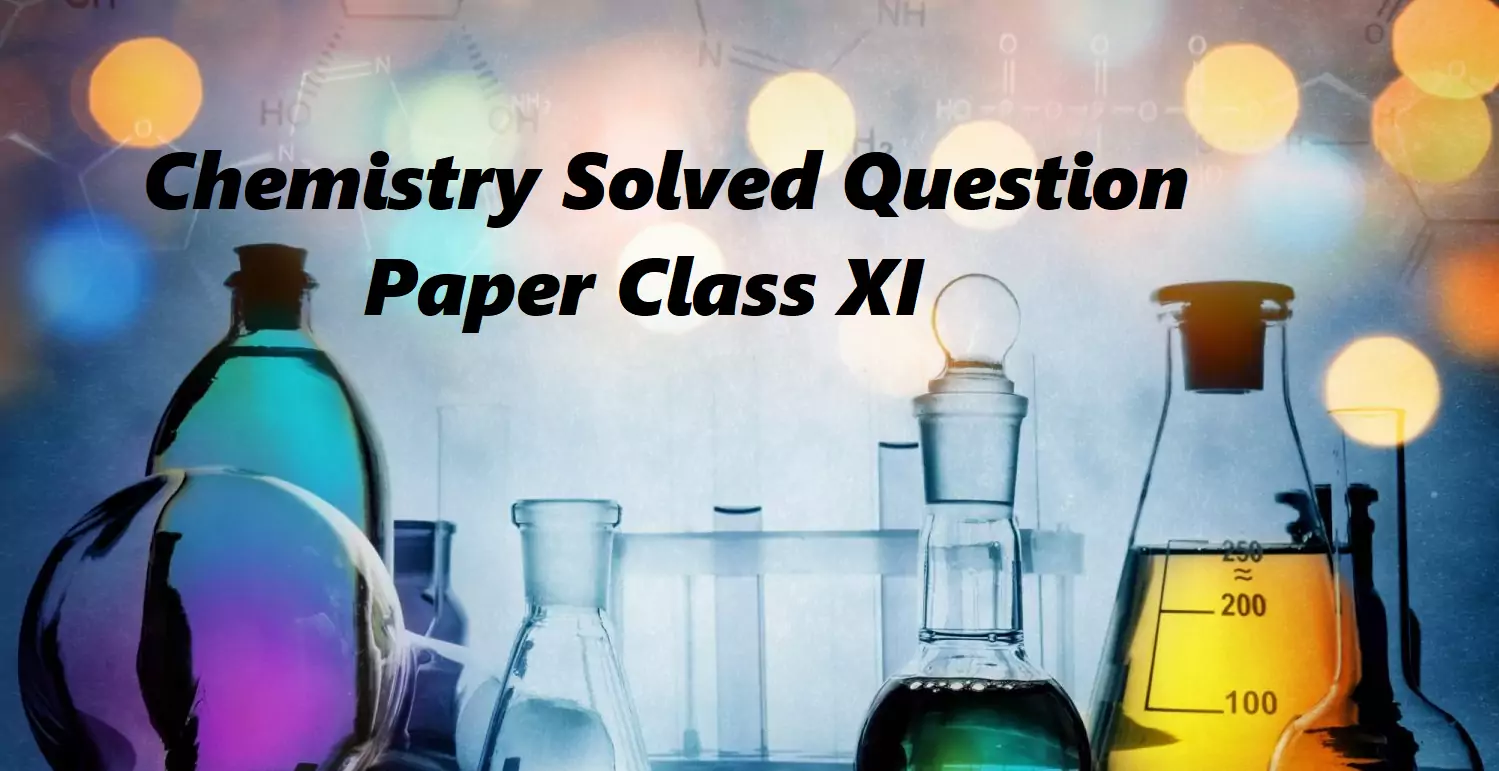Chemistry Solved Question Paper Class XI

### SUBJECT – CHEMISTRY

Class – XI

Session — 2020-2021

Time : 3 hours                                                                                              M.M.-70.

General Instructions:
i. There are 33 questions in this question paper. All questions are compulsory.
ii. Section A: Q.No.1 to 2 are case-based questions having four MCQs or Reason Assertion type based on given passage each carrying 1 mark.
iii. Section A: Question 3 to 16 are MCQs and Reason or Assertion type questions carrying 1 mark each.
iv. Section B: Q.No. 17 to 25 are short answer questions and carry 2 marks each.
v. Section C: Q.No. 26 to 30 are short answer questions and carry 3 marks each.
vi. Section D: Q.No. 31 to 33 are long answer questions and carrying 5 marks each.
vii. There is no overall choice. However, an internal choice has been provided.
viii. Use of calculators and log tables is not permitted.

SECTION A

The modern periodic table arranges the elements in the increasing order of atomic number. It has 18 groups and 7 periods. Atomic numbers are consecutive in a period and increases in the group in a pattern. Elements are divided into four blocks, s-block, p-block, d-block, and f-block based on their electronic configuration. 78% of elements are metals, about 20 elements are non-metals, and few elements like B, Si, Ge, As are metalloids. Metallic character increases down the group but decreases along the period from left to right. The physical and chemical properties vary periodically with their atomic numbers. Periodic trends are observed in atomic size, ionization enthalpies, electron gain enthalpies, electronegativity, and valence. Oxides of metals are basic, some are amphoteric. Non-metals form acidic oxides, some form neutral oxides. s-block elements are soft, highly reactive, do not show variable oxidation states. p-block elements are metals, non-metals as well as metalloids, show variable oxidation states, exist as solids, liquids, and gases. d-block elements are metals, form coloured ions, show variable oxidation states, have high melting and boiling points. Lanthanoids and actinoids are f-block elements, form coloured ions. All actinoids are radioactive.

The following questions are multiple-choice questions. Choose the most appropriate answer:
(a) Name the elements which belong to d-block but are not transition metals.
Ans. Zn, Cd, Hg.

(b) What are representative elements?
Ans. s-block and p-block elements are called representative elements or main group elements.

(c) What is the difference between oxidation states of p-block and d-block elements?
Ans. In p-block elements, oxidation states differ by 2 and the lower oxidation state is more stable where as in d-block, oxidation state differ by 1 and mostly higher oxidation state is more stable.

(d) What happens to reactivity down group 17?
Ans. Reactivity goes on decreasing down the group as a tendency to gain electrons decreases.

Chemistry Solved Question Paper Class XI

In view of the shortcomings of Bohr’s Model of atom, efforts were made to develop a new model of the atom which could overcome the limitations of Bohr’s Model. The development of the new model was mainly based on (i) de Broglie’s concept of the dual nature of matter (ii) Heisenberg’s uncertainty principle.
The new branch of science which was developed taking into consideration the above two concepts is known as Quantum mechanics.

In these questions (Q) No. i-iv, a statement of Assertion followed by a statement of reason is given. Choose the correct answer out of the following choices.

(a). Assertion and reason both are correct statements and reason is the correct explanation for the assertion
(b). Assertion and reason both are correct statements but the reason is not the correct explanation for the assertion.
(c). The assertion is a correct statement but the reason is the wrong statement.
(d). The assertion is the wrong statement but the reason is a correct statement.

(i). Assertion: It is impossible to determine the exact position and exact momentum of an electron simultaneously.
Reason: The path of an electron in an atom is clearly defined

(ii). Assertion: X-ray travel with the speed of light.
Reason: X rays are electromagnetic rays.

OR

Assertion: An electron can never be found in the nucleus.
Reason: Uncertainty in the position of an electron is greater than 10-15m

(iii). Assertion: Mass of moving photon varies inversely as the wavelength.
Reason: Energy of particle=Mass x (speed of light)2

(iv). Assertion: The energy of an electron is mainly determined by the principal Quantum number.
Reason: The energy of an electron is mainly determined by the principal quantum number

Chemistry Solved Question Paper Class XI

Following questions (No.3-11) are multiple-choice questions carrying 1 mark each

3. In which mode of expression, the concentration of a solution remains independent of temperature?
(a) Molarity
(b) Normality
(c) Formality
(d) Molality
Ans 3. (d) Molality

4. How many moles of magnesium phosphate, Mg3(PO4)2 will contain 0.25 mole of oxygen atoms?
(a) 1.25 × 10-2
(b) 2.5 × 10-2
(c) 0.02
(d) 3.125 × 10-2

Ans 4. 1 mole of Mg3(PO4)2 contains 8 moles of oxygen atoms.
Therefore 0.25 moles of oxygen atoms are present in 1/8 × 0.25 moles of Mg3(PO4)2
= 3.125 × 10-2 moles of Mg3(PO4)2

5. Which of the following has more unpaired d-electrons?
(a) Fe2+
(b) Zn+
(c) Ni3+
(d) Cu+
Ans 5. (a) The electronic configurations for various species are
Zn+: 1s2 2s2 2p6 3s2 3p6 4s1 3d10
Fe2+: 1s2 2s2 2p6 3s2 3p6 3d6
Ni3+: 1s2 2s2 2p6 3s2 3p6 3d7
Cu+: 1s2 2s2 2p6 3s2 3p6 3d10

6. The first ionization potential of Na, Mg, Al, and Si are in the order
a) Na < Mg > Al < Si
b) Na > Mg > Al > Si
c) Na < Mg > Al < Si
d) Na > Mg > Al > Si
Ans 6. (a) Across the period the ionization potential increases as the electron enter the same shell so the effective nuclear charge increases thus the order for Na, Al, Si is Na < Al < Si but Mg has the maximum ionization potential because its configuration is 1s2 2s2 2p6 3s2 i.e. completely filled 3s subshell thus more stable have the order is Na < Mg > Al > Si

7. Outermost shell of two elements X and Y have two and six electrons respectively. If they combine, the expected formula of the compound will be
(a) XY
(b) X2Y
(c) X2Y3
(d) XY2
Ans 7: (a) Both elements can show the valency of two. X can lose two electrons to form X2+ whereas Y2– can gain two electrons to form Y. So the formula of the compound formed is XY.

8. The behavior of real gases approach ideal behavior at
(a) low temperature, low pressure
(b) high temperature, high pressure
(c) low temperature, high pressure
(d) high temperature, low pressure
Ans 8. (d) high temperature, low pressure

9. Under which of the following condition is the relation ∆H = ∆U + p∆V valid for a closed system?
(a) Constant Pressure
(b) Constant Temperature
(c) Constant Temperature and Pressure
(d) Constant Temperature, Pressure, and Composition
Ans 9. (a) Constant Pressure

10. Which one of the following is false?
(a). Work is a state function.
(b). Temperature is a state function.
(c). Change in the state is completely defined when the initial and final states are specified
(d). Work appears at the boundary of the system.
Ans 10. (a) A property whose value doesn’t depend on the path taken to reach that specific value is known to as state functions or point functions. Work is a path function. Those functions which do depend on the path from two points are known as path functions.

11. At constant temperature, the equilibrium constant (Kp) for the decomposition reaction N2O4 ↔ 2NO2 is expressed by K = (4x2P)/(1–x2), where P = pressure, x = extent of decomposition. Which one of the following p statements is true?
a. Kp increases with the increase of P
b. Kp increases with the increase of x
c. Kp increases with the decrease of x
d. Kp remains constant with change in P and x.
Ans 11. d. Kp remains constant with change in P and x.

In these questions (Q) No.12-16, a statement of Assertion followed by a statement of reason is given. Choose the correct answer out of the following choices.

12. Assertion (A): Combustion of 16g of methane gives 18g of water.
Reason (R): In the combustion of methane, water is one of the products.

13. Assertion (A): The empirical mass of ethene is half of its molecular mass.
Reason (R): The empirical formula represents the simplest whole-number ratio of various atoms present in a compound.

14. Assertion (A): London forces are attractive forces existing among non-polar molecules or noble gases.
Reason (R): London forces are the attractive forces that operate at all distances between the molecules or atoms.

15. Assertion (A): The temperature at which vapour pressure of a liquid is equal to the atmospheric/external pressure is called boiling temperature.
Reason (R): At high altitude atmospheric pressure is high and so a high boiling point of a liquid is obtained there.

16. Assertion (A): The limiting reagent is the reactant that is entirely consumed. This gives the least amount of product on being entirely consumed.
Reason (R): The reagent which gives the least amount of product on being completely consumed is the limiting reagent.

Chemistry Solved Question Paper Class XI

SECTION – B

The following questions, Q. No. 17-25 are short answer type and carry 2 marks each.

Chemistry Solved Question Paper Class XI

17. Explain why BeH2 has zero dipole moment although Be-H bonds are polar.
Ans 17. The dipole moment of BeH2 is zero because the two equal bond dipoles point in opposite directions and cancel the effect of each other.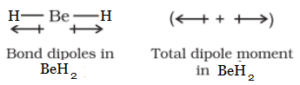OR

Which out of ammonia (NH3) and NF3 has a higher dipole moment and why?
Ans. Out of ammonia (NH3) and NF3, NH3 has a higher dipole moment because, in the case of NH3, the orbital dipole due to lone pair is in the same direction as the resultant dipole moment of the N-H bonds, while in NF3, the orbital dipole is in the direction opposite to the resultant dipole moment of the three N-F bonds. The orbital dipole due to lone pair decreases the effect of the resultant N-F bond moments, which results in the low dipole moment of NF3.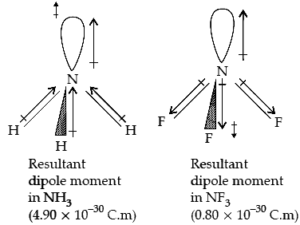18. Using the equation of state PV = nRT shows that at a given temperature density of a gas is proportional to gas pressure p.
Ans. PV = nRT =w​RT/M
Hence, PM = w​RT/V = ρRT
ρ∝P
Here, ρ = w/V​
n = w/M​
ρ is the density, P is the pressure, V is the volume, n is the number of moles, R is the ideal gas constant, T is the temperature and M is the molar mass.

19. Although both CO2and H2O are triatomic molecules, the shape of the H2O molecule is bent while that of CO2is linear. Explain this on the basis of dipole moment.
Ans 19:  In CO2, there are two C=O bonds. Each C=O bond is a polar bond.
The net dipole moment of the CO2 molecule is zero. This is possible only if CO2 is a linear molecule. (O=C=O). The bond dipoles of two C=O bonds cancel the moment of each other.
Whereas, H2O molecule has a net dipole moment (1.84 D). H2O molecule has a bent structure because here the O—H bonds are oriented at an angle of 104.5° and do not cancel the bond moments of each other. Write the electronic configuration of Cr (Z=24) and Cu (Z=29).

20. Out of Na and Mg, which is expected to have higher second ionization enthalpy?
Ans 20. The electronic configuration of both sodium and magnesium are as such:
Na – 2, 8, 1 (3s¹)
Mg – 2, 8, 2 (3s²)
Here, Na has one valence electron in 3s¹, and by losing this one electron it can attain its octet state, thus becoming stable. But if Mg loses one electron, it would still have another electron left in its outermost shell. As a result, the first ionization enthalpy of Na is lower than that of Mg.
Again, now that Na has reached its stable configuration of Neon, Mg has just one electron left in its outermost shell, and by losing it, it will also attain a stable configuration of Neon. But if Na loses one more electron, it will be left with 7 more electrons in it’s L shell, thus becoming unstable once again. As a result, the second ionization enthalpy of Na is higher than that of Mg.

21. Calculate the wave number for the longest wavelength transition in the Balmer series of atomic hydrogen.
Ans 21.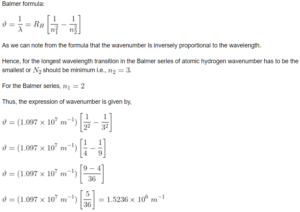Chemistry Solved Question Paper Class XI

22. (a) How will you convert Benzene into
i). Acetophenone
ii). m-nitro chlorobenzene
Ans 22. (i). Benzene reacts with acetyl chloride in the presence of anhydrous AlCl3 to give Acetophenone.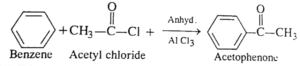(ii). Benzene reacts with conc. HNO3 of conc. H2SO4 to give nitrobenzene which further reacts with chlorine in the presence of anhydrous AlCl3 to give m-nitro chlorobenzene.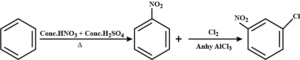(b) Write the structure of the product obtained by ozonolysis of pent-2-ene.
Ans 22 (b).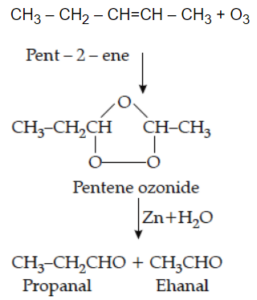OR

Give chemical equations for:
i). Decarboxylation reaction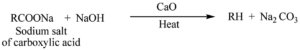ii). Wurtz Reaction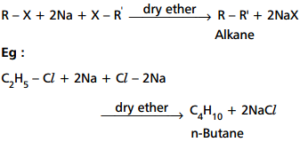iii). Elimination Reaction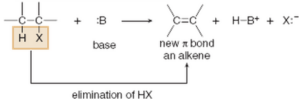23. Give the reason for the following
(a). Boron is unable to form [BF6]-3.
Ans 23 (a). Due to the non-availability of d-orbitals, boron is unable to expand its octet. So, the maximum covalence of boron cannot exceed 4. Hence, boron is unable to form [BF6]-3.
(b). The stability of the +1 oxidation state progressively increases for the heavier element of group 13.
Ans (b). The stability of +1 oxidation state progressively increases for the heavier element of group 13 due to inert pair effect in which on moving down the group, due to poor shielding effect of intervening d and f orbitals, the increased effective nuclear charge holds ns electrons tightly and thereby, restricting their participation in bonding and only p-orbital electron may be involved in bonding.
(c). Graphite is used as a dry lubricant in machines at high temperatures.
Ans (c). Graphite cleaves easily between the layers so it is very soft and slippery. As a result, it is used as a dry lubricant in machines at high temperatures.

24. What will happen when:
(i). Sodium is dropped in water.
Ans (i). When sodium is dropped in water, a vigorous chemical reaction takes place in which sodium hydroxide (NaOH) and hydrogen gas is produced with the evolution of heat.
2Na + 2H2O → 2NaOH + H2
(ii). Sodium metal is heated in a free supply of air.
Ans (ii). When sodium metal is heated in a free supply of air, it reacts vigorously with oxygen to form sodium peroxide.
2Na + O2 → Na2O2

(iii). Sodium peroxide dissolves in water.
Ans (iii). When sodium peroxide dissolves in water, it gives sodium hydroxide and hydrogen peroxide.
Na2O2 + 2H2O → 2NaOH + H2O2

OR

(a) Write down the two uses of Hydrogen peroxide.
Ans (a). Uses of Hydrogen Peroxide-
i). It is used as hair bleach and as a mild disinfectant.
ii). It is used in the synthesis of hydroquinone, tartaric acid, etc.
(b) Calculate the strength of 10 volume solution of Hydrogen peroxide.
Ans (b). 10 volume solution of H2O2 means that 1L of this H2O2 solution will give 10L of oxygen at STP.
2H2O2(l) → O2(g) + H2O(l)
2 × 34                22.7L at
= 68                     STP
∴ 22.7 L of O2 is produced from = 68g H2O2 at STP
∴ 10L of O2 at STP will be produced from
= 29.9
= 30g H2O2
Hence, strength of H2O2 in 10 volume H2O2 solution = 30 g / L = 3% H2O2 solution

25. A compound is composed of 74% C, 8.7% H and 17.3% N. Determine the empirical formula of the compound if the molecular mass of the compound is 162. What Is its molecular formula?
Ans 25. Given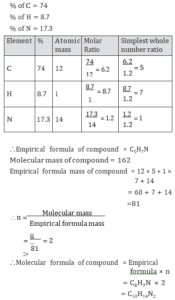Chemistry Solved Question Paper Class XI

26. (a) Write down van der Waal’s equation for ‘n’ mole of gas.
Ans 26 (a). van der Waal’s equation for ‘n’ moles of gas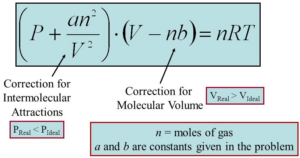(b) At 25°C and 760mm of Hg pressure, a gas occupies 600ml volume. What will be its pressure at a height where temperature Is 10°C and the volume of the gas is 640ml?
Ans (b).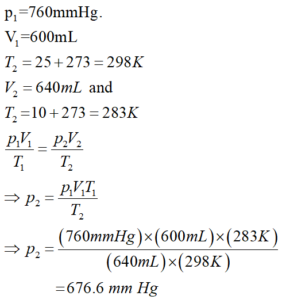27. The concentration of hydrogen ion in a sample of soft drink is 3.8 × 10-3 M. What its pH and predict its nature.
Ans 27. Concentration of H+ ion
[H+] = 3.8 ×10-3M
∴ pH = -log [H+]
= -log[3.8 × 10-3]
= -log 3.8 + 3 log 10
= -0.5798 + 3
= 2.4202
≈ 2.42
∴ pH value of soft drink is less than 7, so it is acidic in nature.

28. (a) State Heisenberg’s Uncertainty Principle.
Ans 28 (a). It states that it is impossible to determine simultaneously the exact position and exact momentum (or velocity) of an electron.
(b) Write down the electronic configuration of Chromium (Z=24).
Ans (b). Electronic configuration of 24Cr
= 2, 8, 13, 1 =1s2, 2s22p6, 3s23p63d5, 4s1
(c) State Hund’s Rule of maximum multiplicity.
Ans (c). It states that pairing of electrons in the orbitals belonging to the same subshell (p, d, or f) does not take place until each orbital belonging to that subshell has got one electron each i.e. it is singly occupied.

29. Assign the reason for each of the following statement:
(a) First ionization enthalpy of Boron (Z=5) is slightly less than that of Beryllium (Z=4).
Ans 29 (a). In beryllium [z = 4, 1s2, 2s2], the electron removed during the ionization is an s-electron while the electron removed during ionization of boron (Z = 5, 1s2,2s2,2p1) is a p-electron. ½
The penetration of a 2s electron to the nucleus is more than that of a 2p-electron, so the 2p electron of boron is more shielded from the nucleus by the inner core of electrons than the 2s electrons of beryllium. Hence, it is easier to remove the 2p- electrons from boron compared to the removal of 2s electrons from beryllium. Hence, the first ionization enthalpy of boron (Z =5) is slightly less than that of beryllium (Z= 4).
(b) Electron gain enthalpy of Fluorine is less negative than that of Chlorine.
Ans (b). Electron gain enthalpy of fluorine is less negative than that of chlorine because when an electron is added to F, the added electron goes to the smaller n = 2 quantum level and suffers significant repulsion from the other electrons present in this level. While for the n=3 quantum level (Cl), the added electron occupies a larger region of space and the electron-electron repulsion is much less.
(c) Size of an anion is always larger than that of the parent atom.
Ans (c). The size of an anion is always larger than that of the parent atom because the addition of one or more electrons would result in increased repulsion among the electrons and a decrease in effective nuclear charge.

30. What will be the pressure of the gaseous mixture when 0.5 L of H2 at 0.8 bar and 2.0 L of dioxygen at 0.7 bar are introduced in a 1L vessel at 27°C?
Ans 30.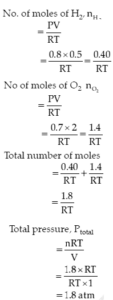Chemistry Solved Question Paper Class XI

Section – D

31. (a) Describe briefly the structure of C2H4 on the basis of hybridization.
Ans 31 (a). The carbon-carbon double bond in ethene (C2H4) consists of one σ – bond due to head-on overlapping of sp2 hybridized orbitals and one π – bond obtained by sideways overlapping of the two 2p orbitals of the two carbon atoms. The structure of C2H4 is shown below: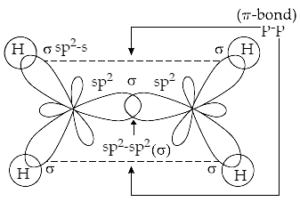(b) Why staggered conformation of ethane is more stable than the eclipsed conformation?
Ans (b). The staggered conformation of ethane is more stable than the eclipsed conformation because, in staggered form, the electron clouds of carbon-hydrogen bonds are as far apart as possible. So, there are minimum repulsive forces.
(c) An unsaturated compound (A) reacts with ozone in presence of zinc dust to give a mixture of propan-2-one and ethanal. Identify (A) and give a reaction.
Ans (c).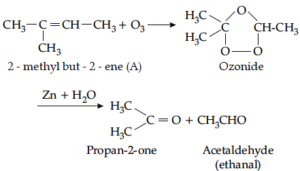OR

(a) Write short notes on (i) Friedel Crafts acylation of Benzene
(ii) Decarboxylation
Ans. (i).(ii).(b) Convert:
(i) Calcium carbide to Benzene
Ans (i).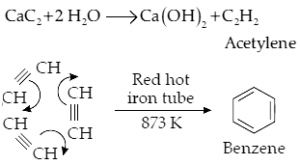(ii) Benzene to Benzene hexachloride (BHC)
Ans.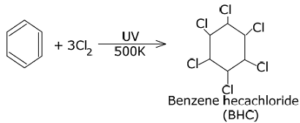(iii) Propene to 1-Bromopropane
Ans.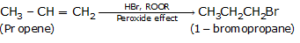32. (i) The ionization of hydrochloric acid in water is given below:
HCl(aq) + H2O(l) → H3O+(aq) + Cl(aq)
Label anyone conjugate acid-base pairs in this ionization.
Ans 32 (i). HCl(aq) +H2O(l) H3O+(aq)  +Cl–(aq)
Conjugate acid-base pair
H2O = Base
H3O+ = Conjugate acid

(ii) Explain the ionic product of water.
Ans (ii). Ionic product of water-
The ionic product of water is defined as the product of the concentration of H+ and OH ions. It is represented by the symbol kw.
Kw = [H+] [OH]

(iii) The degree of ionization of a 0.1M bromoacetic acid solution is 0.132. Calculate the pH of the solution and the pKa of bromoacetic acid.
Ans (iii). Br CH2COOH+H2O Br CH2COO +H3O+

 Initial conc. C 0 0 Equilibrium conc. C-Cα C(1- α ) Cα Cα

Ka = cα. cα/(1- α)
Ka    = Cα2
= 0.1 × (0.132)2
= 0.00174
= 1.74 × 10-3
pKa = – log Ka
= -log [1.74 × 10-3]
= -log 1.74 + 3 log 10
= -2.405+3
= 2.7595
pH= -log [H3O+]
= -log [1.32 × 10-2]
= -log 1.32 +2 log 10
= -0.1206 +2 =1.8794

OR

(i) Oxygen molecule (O2) is paramagnetic. Explain with the help of MOT.
Ans. (i). O2
Total electrons = 8 + 8 = 16
Electronic configuration    😯 = 1s2, 2s2 2p4
8O = 1s2, 2s2 2p4
Molecular orbital configuration,
(σ1s)2,(σ *1s)2,( σ 2s)2,(σ * 2s)2, (σ2pz)2, (π2px)2, (π2py)2, (π*2px)2, (π*2py)1,(σ * 2pz)0
Since, there are two unpaired electrons in molecular orbitals of O2 molecule, so it is paramagnetic in nature.
(ii) On the basis of VSEPR theory, explain the structure of CIF3.
Ans. In ClF3, the central atom Cl contains 7 valence electrons, out of which, three electrons are involved in bonding with three F- atoms, and the remaining four act as two lone pairs of electrons.
bp =3,  lp =2
These 5 electron pairs (3bps +2lps) arrange them in trigonal bipyramidal geometry. But due to lp-lp repulsion, its shape is distorted and becomes T-shape which is shown below33. (a) Gallium has higher ionization enthalpy than aluminium. Why?
Ans. Electronic configuration of
13 Al = 2,8,3
=1s2,2s2,2p6,3s2,3p1
Electronic configuration of
31Ga = 2, 8, 18, 3
= 1s2, 2s2, 2p6, 3s2, 3p6, 3d10, 4s2, 4p1
In gallium, 10 electrons present in 3d sub-shell do not shield the outer electrons from the nucleus effectively. So, effective nuclear charge in Ga increases. That’s why gallium has higher ionization enthalpy than aluminium.

(b) A mixture of dilute NaOH and aluminium pieces is used to open a jammed drain. Why?
Ans. Dilute NaOH reacts with aluminium pieces to form sodium tetrahydroxo aluminate (III) and hydrogen gas. The pressure of hydrogen gas, so produced, is used to open a jammed drain.
(c) What are silicones? How they are prepared?
Ans. They are a group of organosilicon polymers, which have -R2SiO- as a repeating unit. The starting materials for the manufacture of silicones are alkyl or aryl substituted silicon chlorides, RnSiCl(4-n), where R = alkyl or aryl group.
Preparation of silicones:
When methyl chloride reacts with silicon in the presence of copper as a catalyst at 573K, various types of methyl-substituted chlorosilanes are obtained with a small amount of Me4Si. Hydrolysis of dimethyldichlorosilane followed by condensation polymerization forms silicone.

OR

(a) Draw the structure of i) B2H6 ii) SiO44-
Ans.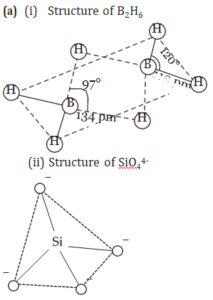(b) A compound A reacts with lithium aluminum hydride (LiAIH4) to form a boron hydride (B). On hydrolysis with water, B forms boric acid and with heating with ammonia, it forms inorganic benzene (C). Identify A, B, and C and write the chemical equations.
Ans (b). Since compound A reacts with   LiAlH4   to form boron hydride. So, compound A may be boron trifluoride and compound B is diborane. Diborane on heating with NH3 to form inorganic benzene (C), which is borazine.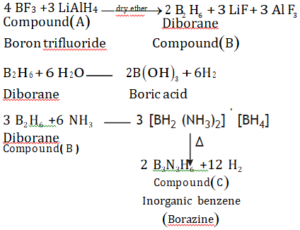CHEMISTRY (CLASS XI)PARAGRAPH BASED QUESTIONS
https://www.sciencemotive.com/class11/chemistry-class-xiparagraph-based-questions/

Chemistry Solved Question Paper Class XI